One of the things we learn in physical and organic chemistry is the state of electron orbitals. By learning how electrons exist, you will be able to understand the state of molecules.

However, the electron states studied in quantum mechanics are abstract and unusually difficult to understand. For example, there are many people who try to study organic chemistry without a deep understanding of the electron orbital state.

However, if you learn about the electron state, you can understand the phenomenon more deeply. Pauli exclusion principle and Hund’s rules are two examples of these electron states.

Some professors may use difficult mathematical equations to explain the Pauli exclusion principle and Hund’s rules. But unless you are a physicist, these formulas are useless. Therefore, we will explain Pauli exclusion principle and Hund’s rules in a simple way without using mathematical formulas, and by comparing them with phenomena in the world.

## The Wave Function in Quantum Mechanics Represents the Probability of the Existence of Electrons

Quantum mechanics is the study of the states of electrons, protons and neutrons. Quantum mechanics typically deals with electrons. The field of quantum mechanics has produced several Nobel Prize winners in physics.

In quantum mechanics, there is something called the wave function. What is the wave function? In high school chemistry, we learn that electrons orbit around atoms like planets.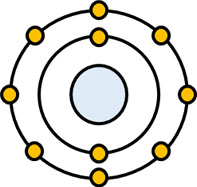But this figure is wrong. The electrons do not revolve around the atoms. The electrons are in orbit, but it is not clear if they exist in an electron orbit. It can be said that there is a high probability that the electron exists in an electron orbit, but it is also possible that the electron exists elsewhere.

The range where electrons exist is called the electron cloud, and the electron orbit is the place where the probability of electrons existing is high. The wave function is what tells us how likely it is that an electron exists in that location.

Electrons are a type of wave, hence the name wave function. It is the wave function that tells us the shape of the electron and the probability of its existence.

## Pauli Exclusion Principle Describes the State of the Electron

So what is the Pauli exclusion principle? Pauli exclusion principle is a branch of quantum mechanics that describes the state of electrons.

Pauli exclusion principle can be described very simply as follows.

• Only two electrons can enter one orbit.

There are different types of electron orbitals. The s and p orbitals are particularly important in physical and organic chemistry. They look like the following.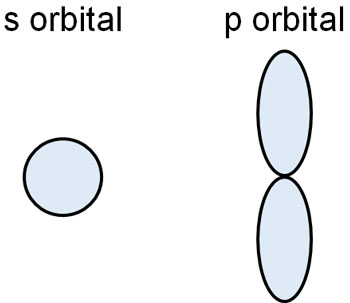Electrons have a high probability of existing in these orbitals. However, electrons are not free to enter these orbitals; only a maximum of two electrons can enter a single orbit. This is the Pauli exclusion principle.

In 1945, the Swiss physicist Wolfgang Pauli was awarded the Nobel Prize in Physics. He was awarded for his discovery of the Pauli exclusion principle.

### Electrons Have a Spin, and They Have to Face Each Other in Opposite Directions

There are other important ideas in the Pauli exclusion principle. It is the orientation of the electron. When the electrons enter the orbit, they must be in a specific direction. They must be facing away from each other to enter the electron orbit.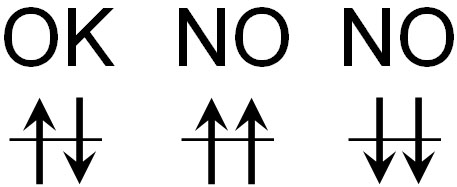Electrons have a spin. Electrons also have an electric charge. When an electrically charged substance rotates, it produces a magnetic force.

Magnets have an N-pole and an S-pole, and if they go in the same direction, they repel. For example, if you put them close to the N-pole and the N-pole, they will repel. In order to cancel each other out, electrons enter opposite directions in the electron orbits, not in the same direction.

To be more precise, electrons with the same wave function cannot enter the same orbit. But using the term “wave function” is difficult to understand. So, let’s understand that electrons spinning in the same direction cannot enter the same electron orbit.

### Examples of Pauli Exclusion Principle in Our Daily Life

The Pauli exclusion principle is all around us. For example, phosphorescence is one such example. The following is a road that absorbs the sun’s rays during the day and emits light at night.Phosphorescence is the phenomenon of continuously emitting weak light, even when we stop shining light on it. Why does phosphorescence continue to emit light even when the light is not shining on it, unlike fluorescence? This has to do with the Pauli exclusion principle.

When light energy strikes an electron, the electron moves to another orbit; this is called electronic transition. However, the state in which the electron transition occurs is unstable (excited state) and tries to return to the original state (ground state). At this time, the electrons return to the ground state by releasing heat and light.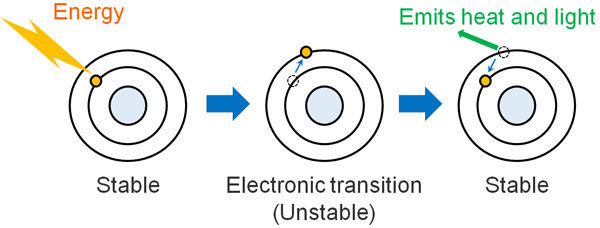In some materials, however, the electron spin can change direction after the electron transition when light hits the material. In technical terms, this state is called a triplet excited state.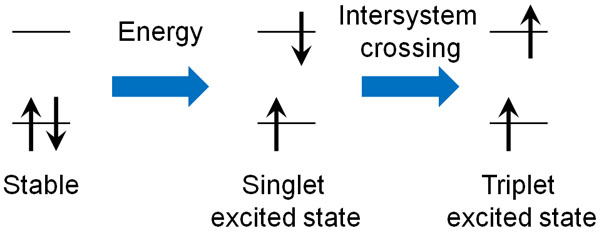According to the Pauli exclusion principle, electrons with the same wave function (electron spins) cannot enter the same orbit.

After the electron transition, the direction of the electron spins must be reversed in order to return to the ground state from the unstable excited state. Therefore, the electron cannot return to the ground state immediately from the excited state and emits weak light continuously for a long time. This is the principle of phosphorescence.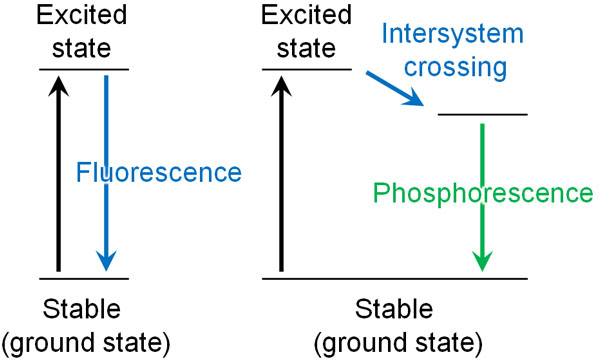If we learn the Pauli exclusion principle, we can understand why phosphorescence occurs. Some of the phenomena around us can be explained by the Pauli exclusion principle.

It is known that the Pauli exclusion principle applies to not only electrons but also protons and neutrons without exception. Although there are some exceptions, many substances follow the Pauli exclusion principle.

## Hund’s Rules to Learn Electronic Placement

Does an electron orbit always have to have two electrons? Of course not. When two electrons are in the same electrical orbit, the electron density increases. The electrons should be separated from each other because they repel each other.

In such cases, Hund’s rules are used to indicate the order in which the electrons enter the orbit. Hund’s rules are the rule for electron placement. Hund’s rules are as follows.

• When electrons enter an orbital, they enter the different orbitals in order.

Each molecule has its own orbitals. If there are orbitals of the same energy, then when the electrons enter the orbitals, they will enter different orbitals one by one. This is Hund’s rules.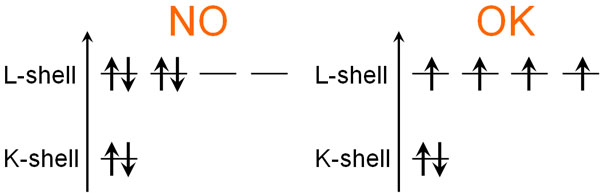Thus, one by one, the electrons go in.

### The Electrons Enter in Order of Lowest Energy Orbitals

But the electron configuration we just described is incorrect because it doesn’t take into account the s and p orbitals. There are two types of electron orbitals, s-orbitals and p-orbitals, and when comparing the energies of the two, the s-orbitals have lower energy.

Even in the same L-shell, the energy of the s-orbital is lower than that of the p-orbital. As a result, two electrons enter the s orbital first. After that, they go into the p orbitals one by one. For carbon, nitrogen, and oxygen atoms, Hund’s rules state that they enter the electron orbitals as follows.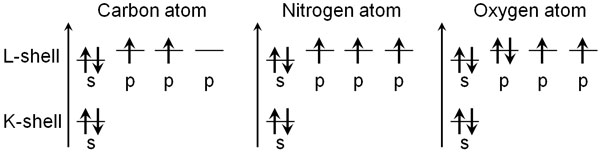Each atom has the following electrons.

• Carbon atom: 6
• Nitrogen atom: 7
• Oxygen atom: 8

If the electrons enter in the order of low energy, the figure above shows the result. Since the energy is lower in the s-orbital, two electrons initially enter the s-orbital. After that, the electrons enter the p-orbital, which has three orbitals with the same energy. Therefore, when the electrons enter the p orbitals, they enter each orbit one by one.

The electron configuration follows these rules. When the electrons are arranged according to Hund’s rules, unpaired electrons are created. In addition to that, the number of unpaired electrons is maximized.

In any case, this electron configuration of the atoms is Hund’s rules. By learning Hund’s rules, we can understand the electron configuration of atoms, their energies, and the bonds between molecules.

## Understanding the Environment of Electrons in Atoms and Molecules

An important element in chemistry is the state of electrons. If we know what state the electrons are in, we can understand how chemical reactions work.

However, the difficulty with these quantum mechanics is that they cannot be clearly identified as in organic chemistry or biochemistry. For example, in a biological experiment, we can check the state of cells. On the other hand, it is difficult to identify the electrons in atoms and molecules.

Therefore, it is inevitably difficult to understand the wave function in quantum mechanics. There is the Pauli exclusion principle and Hund’s rules in such quantum mechanics.

However, the concepts of Pauli exclusion principle and Hund’s rules are simple if you don’t use difficult mathematical formulas. These laws are the principles that describe the state and arrangement of electrons.

If you want to know why these laws are valid, you will need to do difficult calculations. In that case, you will need to study physics in depth as quantum mechanics. However, it is not necessary for many people to do difficult calculations to know the reasons, so let’s understand the outline of these laws.